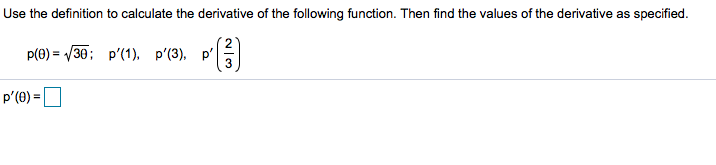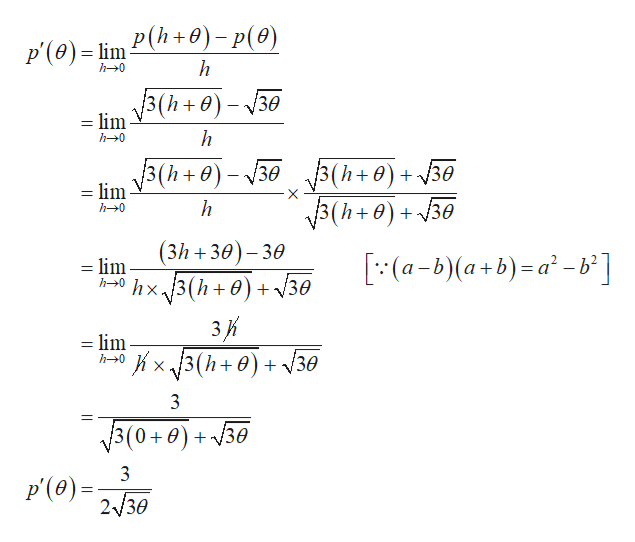specifiedUse the definition to calculate the derivative of the following function. Then find the values of the derivative asp(Ө) —D 30; р'(1). р'(3), р'p'(e)

Question

Can you please help me step by step with this problem?help_outlineImage Transcriptionclosespecified Use the definition to calculate the derivative of the following function. Then find the values of the derivative as p(Ө) —D 30; р'(1). р'(3), р' p'(e) fullscreen
Step 1

Derivative can be compu...help_outlineImage TranscriptioncloseP'(ө) - im P(h+0) - p(ө) h h-0 = lim V3(h+)-30 h h0 3(h+0)-30(h+)+30 =lim 3(h+0) + 30 3(h+0)+30 h h0 (3h 30)-30 (a-b)(a+b)-a-b] = lim h0hx.13(h+ 0) + /30 3К =lim x3(h+0)30 h0 3 3(0+0) + у30 3 p(0)230 fullscreen

Want to see the full answer?

See Solution

Want to see this answer and more?

Our solutions are written by experts, many with advanced degrees, and available 24/7

See Solution
Tagged in

Calculus